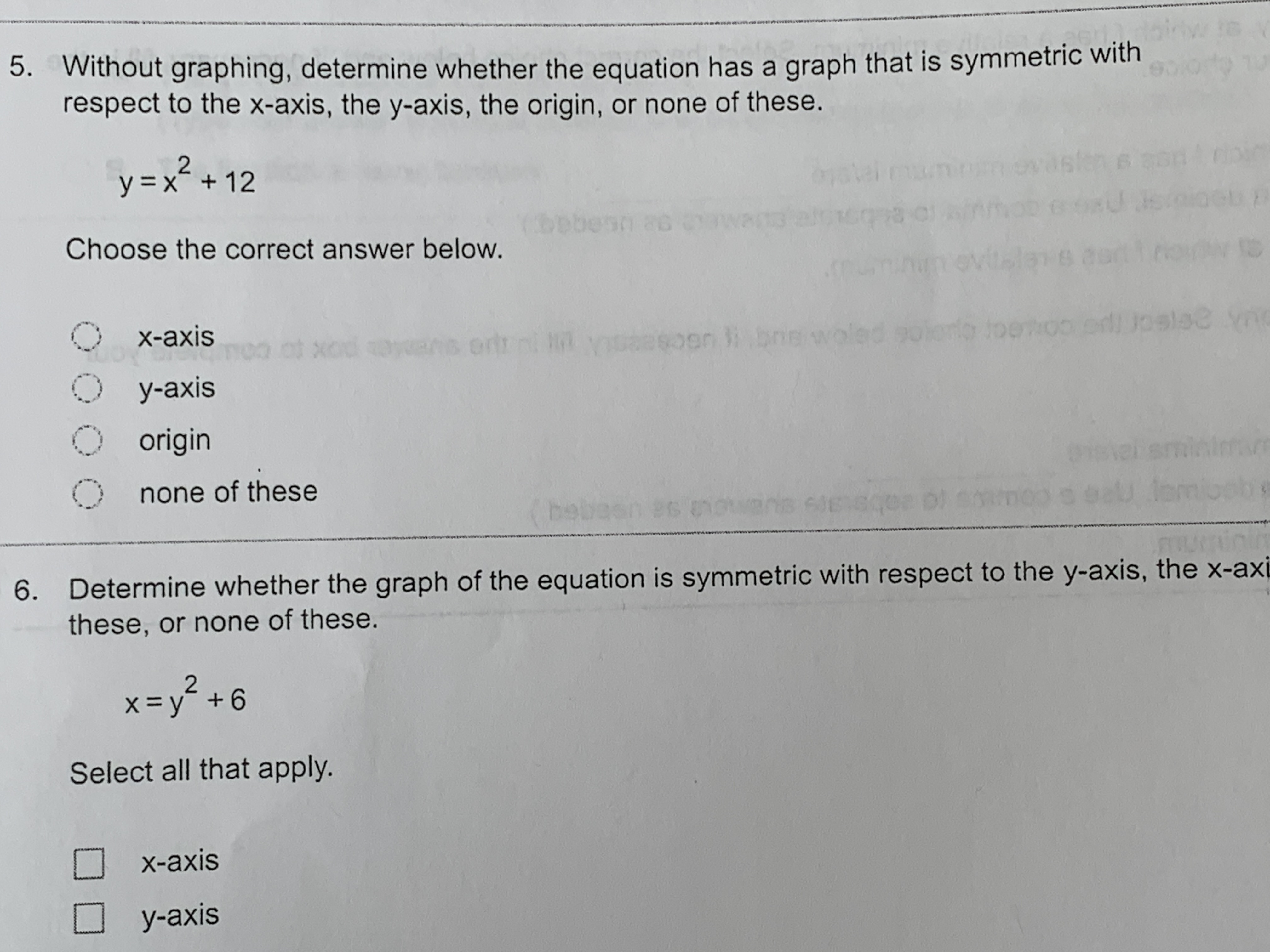# Without graphing, determine whether the equation has a graph that is symmetric wit respect to the x-axis, the y-axis, the origin, or none of these. 5. stn e 2 y x12 72 28 Choose the correct answer below. 1 8 leC oedoo ed! Uoeloe yAe 50 X-axis o l bre ort i X00 3 8 O y-axis 0 origin 1s Onone of these 6UB Determine whether the graph of the equation is symmetric with respect to the y-axis, the x-axi these, or none of these. 6. 2 =y +6 Select all that apply. x-axis y-axis

Question

Can someone help me solve this problem? It is number fivehelp_outlineImage TranscriptioncloseWithout graphing, determine whether the equation has a graph that is symmetric wit respect to the x-axis, the y-axis, the origin, or none of these. 5. stn e 2 y x12 72 28 Choose the correct answer below. 1 8 leC oedoo ed! Uoeloe yAe 50 X-axis o l bre ort i X00 3 8 O y-axis 0 origin 1s Onone of these 6UB Determine whether the graph of the equation is symmetric with respect to the y-axis, the x-axi these, or none of these. 6. 2 =y +6 Select all that apply. x-axis y-axis fullscreen

### Want to see this answer and more?

Experts are waiting 24/7 to provide step-by-step solutions in as fast as 30 minutes!*